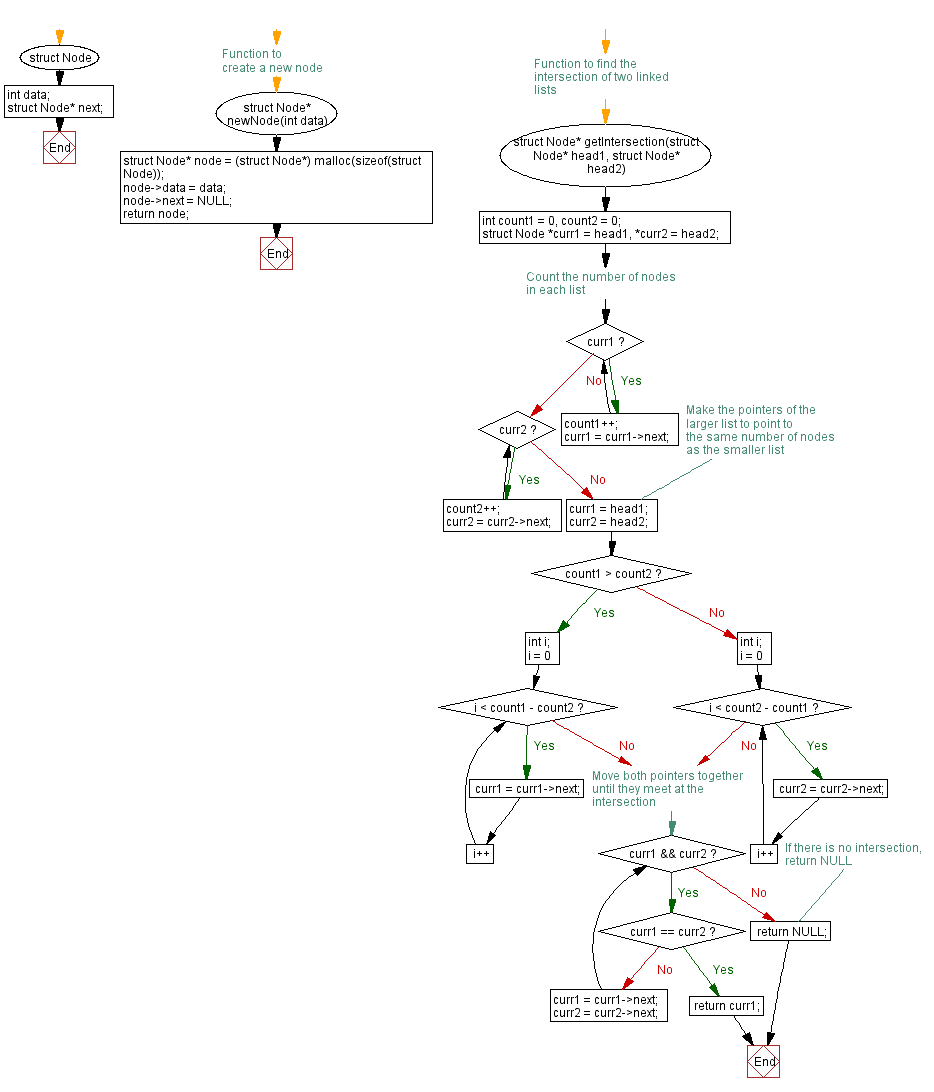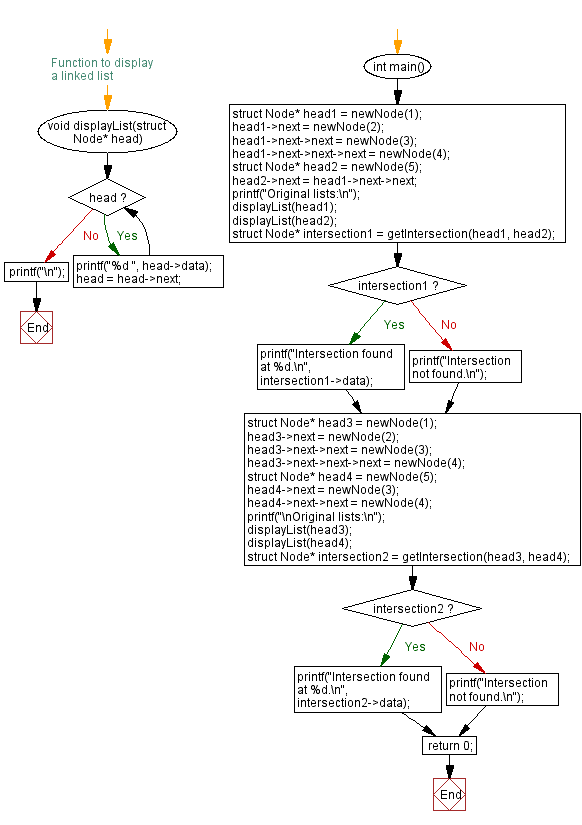﻿ C Program: Find the intersection of two singly linked lists

# C Exercises: Find the intersection of two singly linked lists

## C Singly Linked List : Exercise-19 with Solution

Write a C program to find the intersection of two singly linked lists.

Sample Solution:

C Code:

``````#include<stdio.h>
#include <stdlib.h>

struct Node {
int data;
struct Node* next;
};

// Function to create a new node
struct Node* newNode(int data) {
struct Node* node = (struct Node*) malloc(sizeof(struct Node));
node->data = data;
node->next = NULL;
return node;
}

// Function to find the intersection of two linked lists
int count1 = 0, count2 = 0;

// Count the number of nodes in each list
while (curr1) {
count1++;
curr1 = curr1->next;
}
while (curr2) {
count2++;
curr2 = curr2->next;
}

// Make the pointers of the larger list to point to the same number of nodes as the smaller list
if (count1 > count2) {
int i;
for (i = 0; i < count1 - count2; i++)
curr1 = curr1->next;
} else {
int i;
for (i = 0; i < count2 - count1; i++)
curr2 = curr2->next;
}

// Move both pointers together until they meet at the intersection
while (curr1 && curr2) {
if (curr1 == curr2)
return curr1;
curr1 = curr1->next;
curr2 = curr2->next;
}

// If there is no intersection, return NULL
return NULL;
}

// Function to display a linked list
}
printf("\n");
}

int main() {

printf("Original lists:\n");

if (intersection1)
printf("Intersection found at %d.\n", intersection1->data);
else

printf("\nOriginal lists:\n");

if (intersection2)
printf("Intersection found at %d.\n", intersection2->data);
else

return 0;
}
```
```

Sample Output:

```Original lists:
1 2 3 4
5 3 4
Intersection found at 3.

Original lists:
1 2 3 4
5 3 4
```

Flowchart :C Programming Code Editor:

What is the difficulty level of this exercise?

﻿